# Random space filling of the plane

Written by Paul Bourke
July 2011

Initial concept and inspiration by John Shier.
Additional examples by John Shier: statistical_geometry_examples.pdf

The following illustrates a technique of iteratively tiling the plane with non-overlapping shapes where, on each iteration, the position is determined randomly and the area is some decreasing function. Note that the shapes, when circles, are not added as "Soddy" circles as in Apollonian  space filling fractals, nor do they even need to touch at a single point of another shape . If the area of the initial shape is A0 and the decreasing function at iteration i is g(i) then the area at each iteration is :

A0, A0 g(1), A0 g(2), .... A0 g(n)

The total area at the n'th iteration is given by the following, and it is this area that needs to match the bounded area of the plane being filled.

 n-1 An = A0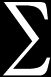g(i) i=0

There are many possible choices for g(i), but choices that are space filling are possibly very few . The choice here is as follows where "c" is some constant.

 1 g(i) =i c

If the function g(i) decrease too fast the space cannot be filled (left image below), if it doesn't decrease fast enough there isn't enough space to add the next shape (right image below) and satisfy the non-overlapping criteria.g(i) decreases too fastg(i) decreases too slowely

The total area for the infinite series is then given by :Atotal = A0i -c i=0

Which is known as the Riemann zeta function  which converges for c > 1. So, for space filling one can choose a value of "c" which then dictates the value of A0. If one chooses a value of "c" that is greater than around 1.5 then the area of the first shape usually becomes too large for "interesting" tilings.

The algorithm used to create the images on this page involves choosing a value of "c" and then calculating the value of A0. A potential placement for the next (i'th) shape is made randomly (uniform distribution). If this candidate position results in an overlap with a shape already on the plane then another potential random placement is tried. This process is repeated until a non overlapping position is found at which point the shape is placed on the plane.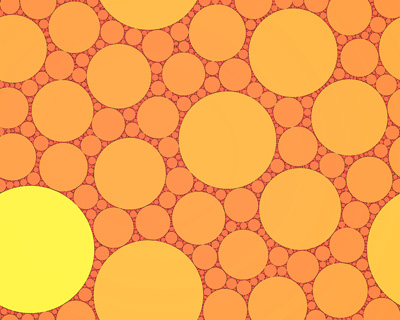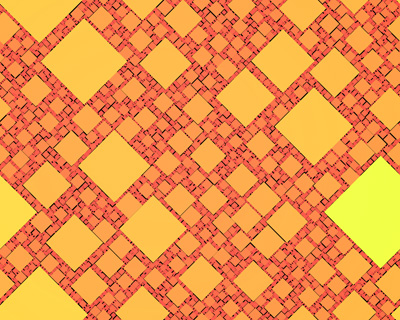Pseudo-code might be as follows

 ```choose value of c calculate initial area from Riemann zeta relationship initiate random number generator repeat for i=0 to some chosen number of iterations n area of new object = initial area multiplied by pow(i,-c) calculate the dimensions of the new object given the area of the new object repeat choose a random position in the region of the plane being filled check for intersection of the new object at this position with all other objects if the new object does not intersect exit the repeat loop end repeat add the new new object to the plane end repeat ```

Periodic, also known as toroidal bounds, results in a rectangular region that can seamlessly tile the entire plane. This is implemented by checking if the boundary of the shape to be added extends past the bounds of the rectangular region, if it does then additional objects are added +- the width and/or +- the height of the rectangle.Any geometric primitive can be used as long as one can calculate the area and perform a test whether two primitives intersect. This test is straightforward for some shapes (eg: circle, rectangle) and a little more involved for other shapes. The intersection tests for polygons involves testing whether any vertex of one polygon is inside the other and whether any edges intersect. While there are special (simpler) tests for triangles and rectangles using the general polygon intersection test is used here for rotated versions of the triangle and rectangle.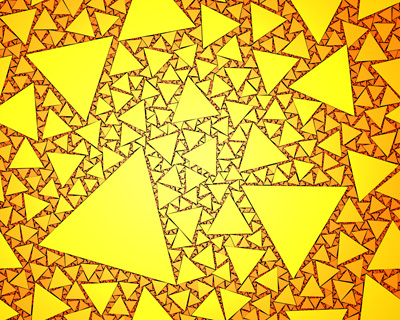When comparing any two objects for intersection the first test is to see if the bounding circles intersect, this is a simple comparison of the sum of the two radii and the distance between the two objects.

```int Separated(XY p1,XY p2,double r1,double r2)
{
double dx,dy,dr;

dx = p1.x - p2.x;
dy = p1.y - p2.y;
dr = sqrt(dx*dx + dy*dy);
if (dr > r1 + r2)
return(TRUE);
else
return(FALSE);
}
```

The general intersection test between two polygons is as follows:

```/*
Return FALSE if two polygon intersect.
Polygon p1[] is of length n1 and polygon p2[] is of length n2.
Polygons are define with vertices ordered clockwise.
*/
int PolygonPolygon(XY *p1,XY *p2,int n1,int n2)
{
int i,j;

// Reject if a vertex of p1 is inside p2, And visa versa
for (i=0;i<n2;i++) {
if (InsidePolygon(p1,n1,p2[i]))
return(FALSE);
}
for (i=0;i<n1;i++) {
if (InsidePolygon(p2,n2,p1[i]))
return(FALSE);
}

// Reject any intersecting edges
for (j=0;j<n1;j++) {
for (i=0;i<n2;i++) {
if (LineIntersect(p1[j].x,p1[j].y,p1[(j+1)%n1].x,p1[(j+1)%n1].y,
p2[i].x,p2[i].y,p2[(i+1)%n2].x,p2[(i+1)%n2].y))
return(FALSE);
}
}

return(TRUE);
}
```

The supporting functions for these tests are given here: LineIntersect.c and here: InsidePolygon.c.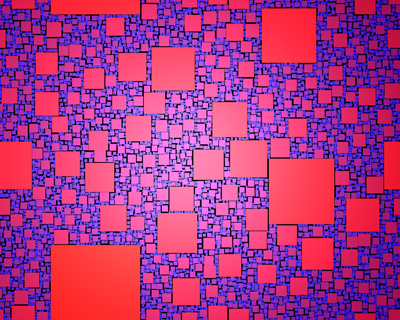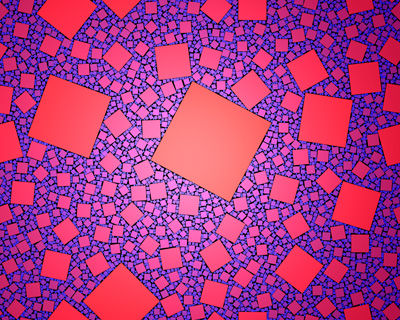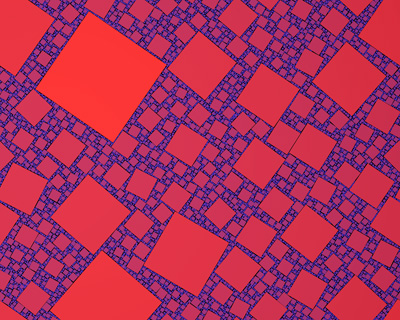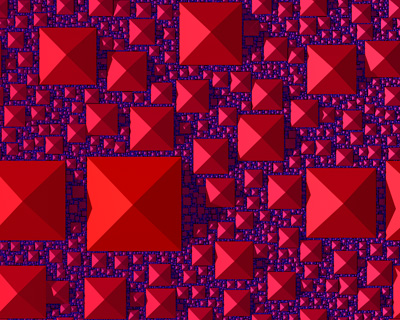The following is an estimate for the fractal dimension of the negative space from the circular object case. This has been calculated for two cases, a 90% fill and the first 10000 disks. The algorithm used employed offset origins in order to calculate the minimum coverage, this results in a much higher quality estimate of fractal dimension and described here.The extension into 3D is relatively straightforward but it then becomes a challenge to visualise. A simpler extension which might be called 2.5D is to use 3D objects that have the same cross-section as the 2D shape being tiled. It additionally helps if the cross-sections in height are decreasing functions in radius.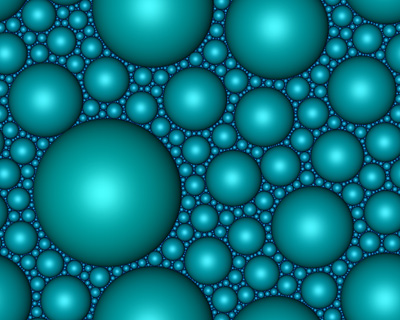References

1. John Shier. Hyperseeing, summer 2011 issue, pp. 131-140, published by ISAMA (International Society of the Arts, Mathematics, and Architecture). Filling Space with Random Fractal Non-Overlapping Simple Shapes

2. Abramowitz and Stegun. Handbook of Mathematical Functions.

3. Paul Bourke. Apollony fractal. Computers and Graphics, Vol 30, Issue 1, January 2006, pages 134-136.

4. S.S. Manna. Space filling tiling by random packing of discs. Physica A: Statistical Mechanics and its Applications Volume 187, Issues 3-4, 15 September 1992, Pages 373-377.

5. Andrew F. Siegel. Random Space Filling and Moments of Coverage in Geometrical Probability. J. Appl. Prob. 15, pp340-355, 1978.

6. R. Mahmoodi Baram, Hans J. Herrmann. Random Bearings and their Stability. Physical Review Letters, Vol.95, 224303 (2005).

7. John Shier. Statistical Geometry. http://www.john-art.com

8. Clifford A, Pickover. Cleopatra's necklace and the aesthetics of oscilatory growth. The Visual Computer, Vol 9, No 3 pp 166-169.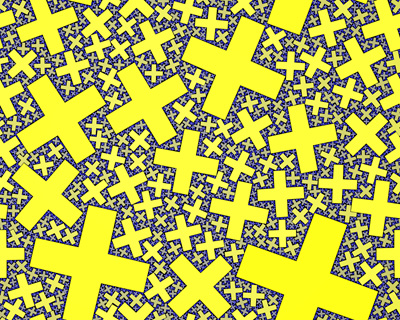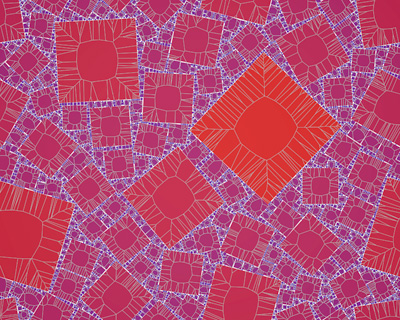Voronoi diagram### Extension to 3D

While in some of the examples above a 3D primitive was used, it was only the cross section through the plane that contributed to the tiling. Extending this to packing in 3D is straightforward, the main difference is that it is harder to present and visualise the results. With regard to the theory discussed at the start of this document, one simply replaces areas with volumes, the same function g(i) is used to determine the volume of the object added at iteration i. As discussed earlier for whichever shape one is using for the volume filling, one needs to be able to perform an intersection test between the position of the proposed object and all the current objects. As before, this test is the simplest for a sphere and simple for axis aligned boxes.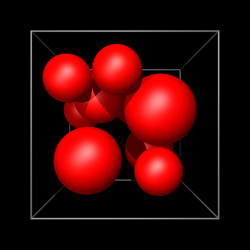10 spheres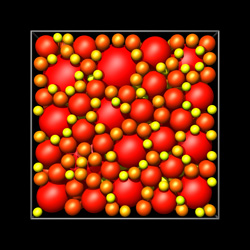100 spheres10,000 spheres

For objects formed by facets (mesh), the intersection test is more involved. The comparison between two objects starts with the test of whether their bounding spheres intersect. Each vertex of one shape is then tested to see if it lies within the other shape. For convex shapes this can be achieved by testing if the vertex is always on the same side of the facets (assuming they are ordered consistently). For more general shapes one can count the intersections of a line from the vertex being tested to infinity on the x axis. If this count is even then the vertex is on the exterior, if odd it is on the interior. And finally one tests to see if the edges of each shape intersect the facets of the other shape.100 cubes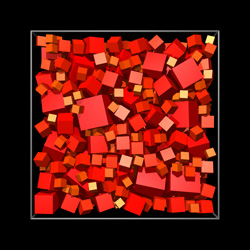1,000 cubes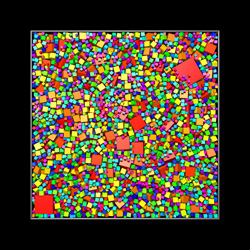35,000 cubes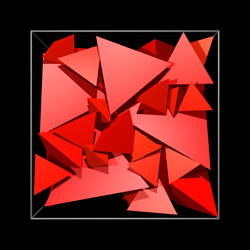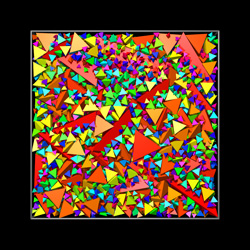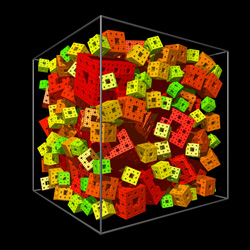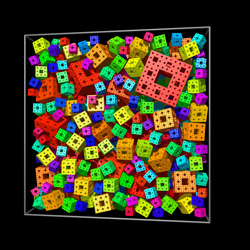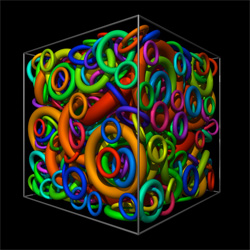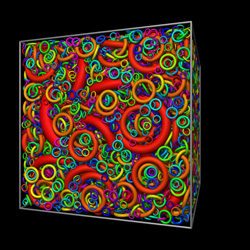The algorithm is not limited to filling rectangular regions, the value of "c" only depends on the total area to be filled, not the shape.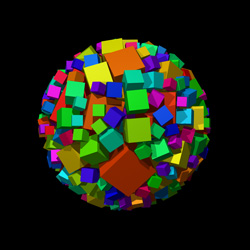Spherical boundary conditions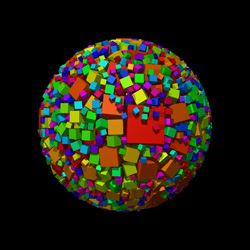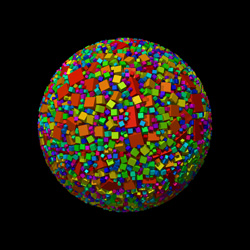10,000 cubes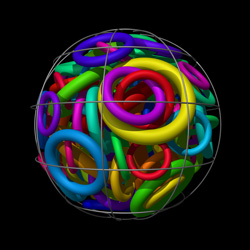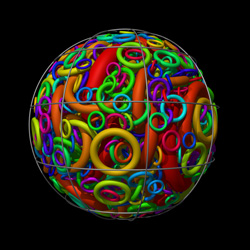### 1 dimensionEstimates of fractal dimension of the dust (holes in the above figures) as a function of "c" are shown below. The four curves give estimates for two different fill percentages and two different segment counts. These are computed by the 1 dimensional equivalent to 2D box counting, finding the minimum coverage count N across a range of ruler lengths "s" and then taking the slope of the log(N) and log(1/s) graph.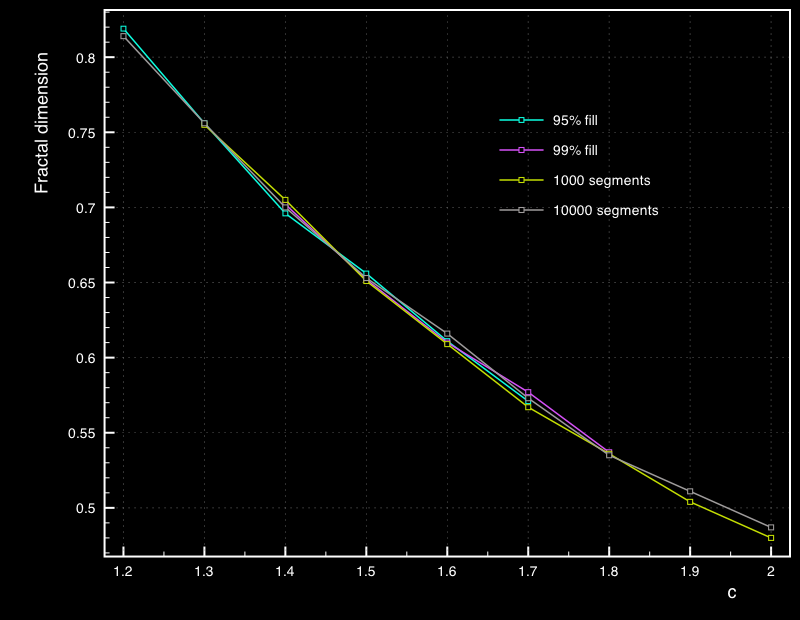Zoom in 2 Dimensions based upon 1 million spheres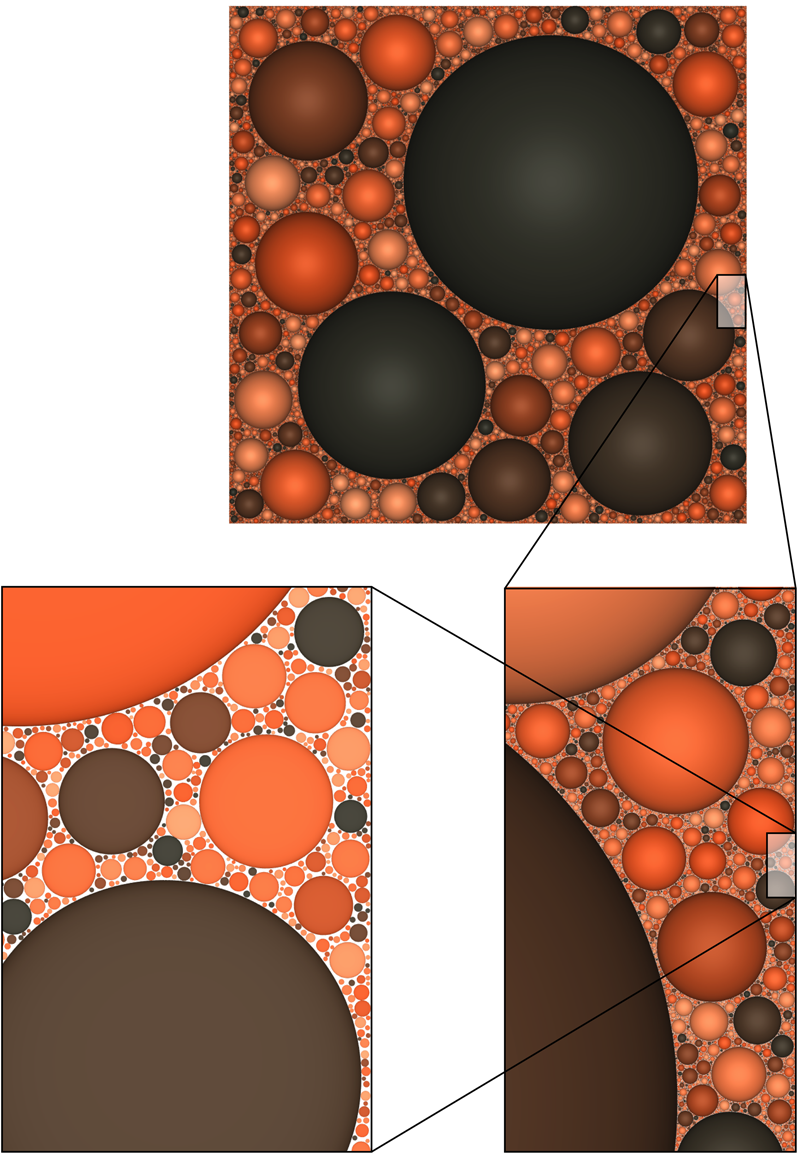Pawsey Supercomputer Centre Entrance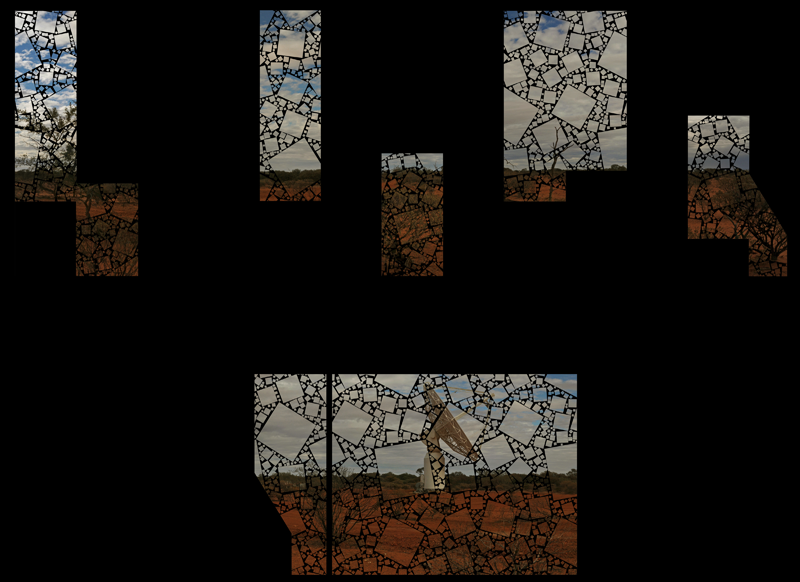Flow disgram